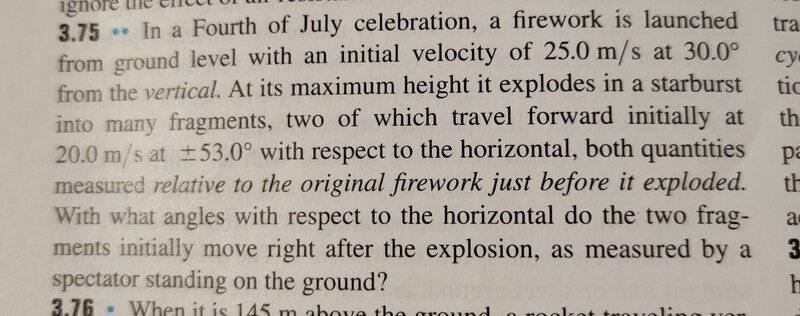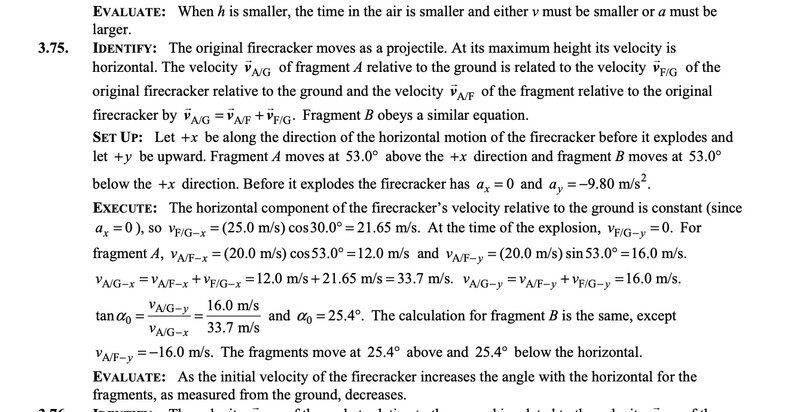# Relative Velocity and Angles of Movement (Sears & Zemansky's Exercise)

• Argonaut
I'm just a little confused as to why the problem statement would specify "30.0° from the vertical" if they meant horizontal. Seems like a careless mistake.Thanks for...well, the confirmation mostly. I'm just a little confused as to why the problem statement would specify "30.0° from the vertical" if they meant horizontal. Seems like a careless mistake.In summary, the conversation was about a problem where a firework explodes into fragments and the question is about finding the angles at which two fragments move with respect to the horizontal, as measured by a spectator standing on the ground. The official solution gives an answer of ±25.4°, but the person discussing the problem is having trouble

#### Argonaut

Homework Statement
In a Fourth of July celebration, a firework is launched from ground level with an initial velocity of 25.0 m/s at 30.0° from the vertical. At its maximum height it explodes in a starburst into many fragments, two of which travel forward initially at 20.0 m/s at ±53.0° with respect to the horizontal, both quantities measured relative to the original firework just before it exploded. With what angles with respect to the horizontal do the two fragments initially move right after the explosion, as measured by a spectator standing on the ground?
Relevant Equations
Basic trigonometry
The official solution says ±25.4°, but I'm having trouble reproducing it. Here is my solution:

1) The components of the velocity of firework F with respect to the ground G in the moment of explosion are the following (Notice, I'm using sin, because the statement says 30.0° from vertical.)

$$v_{F/G-x}=\sin{30,0^{\circ}} * 25.0 \textrm{m}/\textrm{s}=12.5\textrm{m}/\textrm{s}$$

$$v_{F/G-y}=0$$

2) The components of the velocity of the piece of shrapnel S are the following (for the sake of simplicity, observing only the one flying above the horizontal):

$$v_{S/G-x}=v_{S/F-x}+v_{F/G-x}=(\cos{53,0^{\circ}} * 20.0 \textrm{m}/\textrm{s}) + 12.5\textrm{m}/\textrm{s} = 24.5 \textrm{m}/\textrm{s}$$

$$v_{S/G-y}=v_{S/F-y}+v_{F/G-y}=(\sin{53,0^{\circ}} * 20.0 \textrm{m}/\textrm{s}) + 0=16.0\textrm{m}/\textrm{s}$$

3) Finding the angle:

$$\alpha = \tan^{-1}{(v_{S/G-y}/v_{S/G-x})} = 33.1^{\circ}$$

Therefore, the two pieces of shrapnel move at ±33.1° with respect to the horizontal and as measured by a spectator standing on the ground.

Except that the official solution says ±25.4°
, which is the value I get if at step 1, I calculate the x component of the velocity of the firework using the cosine and not the sine, so if ##v_{F/G-x}=\cos{30,0^{\circ}} * 25.0 \textrm{m}/\textrm{s}=21.65\textrm{m}/\textrm{s}##. But the statement says 30.0° from vertical.

Am I misunderstanding what 'from vertical' means? Or have I made some other trig error?

•PeroK
Well reasoned, well explained, well calculated, well presented. And with good reverse engineering of the book answer.

Nicely done.

•PhDeezNutz, PeroK and Argonaut
jbriggs444 said:
Well reasoned, well explained, well calculated, well presented. And with good reverse engineering of the book answer.

Nicely done.
Does this mean the book answer is wrong, you reckon?

Argonaut said:
Does this mean the book answer is wrong, you reckon?
Yes, the book is wrong. It will not be the first time. Nor the last.

jbriggs444 said:
Yes, the book is wrong. It will not be the first time. Nor the last.
Thanks! I thought I was going mad, but it was good practice at least.

Argonaut said:
Thanks! I thought I was going mad, but it was good practice at least.
hei sir.i'm sorry to say maybe the book is right.
maybe firework with an initial velocity of 25.0 m/s at 30.0° with respect to the horizontal.
and it was calculated that the answer is 25.4

•PeroK
YanZhen said:
hei sir.i'm sorry to say maybe the book is right.
maybe firework with an initial velocity of 25.0 m/s at 30.0° with respect to the horizontal.
and it was calculated that the answer is 25.4
Hello! I've copied the homework statement word for word, and it explicitly says "30.0° from the vertical".

I located the exercise on the web and can confirm:
3.75 .. In a Fourth of July celebration, a firework is launched from ground level with an initial velocity of at 30.0° from the vertical. At its maximum height it explodes in a starburst into many fragments, two of which travel forward initially at at with respect to the horizontal, both quantities measured relative to the original firework just before it exploded. With what angles with respect to the horizontal do the two fragments initially move right after the explosion, as measured by a spectator standing on the ground?

[There are quite a few hits. None of the ones I looked at are Sears and Zemansky, but none say 30 degrees from the horizontal]

Last edited:
It's the 13th edition of Sears and Zemansky's University Physics:•topsquark and jbriggs444
Argonaut said:
It's the 13th edition of Sears and Zemansky's University Physics:
View attachment 315962
then,either the question or the answer is wrong.

@Argonaut I did the problem myself and got the same answer as you. As you mentioned in the OP you get the book answer if you choose ##30 ^{\circ}## to be from the horizontal (instead of the vertical as stated in the problem)

Looking at the instructor's solution manual (which I will not distribute) they also chose ##30 ^{\circ}## to be from the horizontal (instead of the vertical as stated in the problem) and that is how they got to an answer of ##\theta = 25.4 ^{\circ}##.

So your answer is right based on the question given. Theirs (the solution manual's) is not. When a person is tasked with solving hundreds to thousands of problems they are bound to make mistakes.

You are right, and good on you for checking the alternate scenario when you felt something was off.•YanZhen and Argonaut
PhDeezNutz said:
@Argonaut I did the problem myself and got the same answer as you. As you mentioned in the OP you get the book answer if you choose ##30 ^{\circ}## to be from the horizontal (instead of the vertical as stated in the problem)

Looking at the instructor's solution manual (which I will not distribute) they also chose ##30 ^{\circ}## to be from the horizontal (instead of the vertical as stated in the problem) and that is how they got to an answer of ##\theta = 25.4 ^{\circ}##.

So your answer is right based on the question given. Theirs (the solution manual's) is not. When a person is tasked with solving hundreds to thousands of problems they are bound to make mistakes.

You are right, and good on you for checking the alternate scenario when you felt something was off.
Thanks for confirming.

•PhDeezNutz